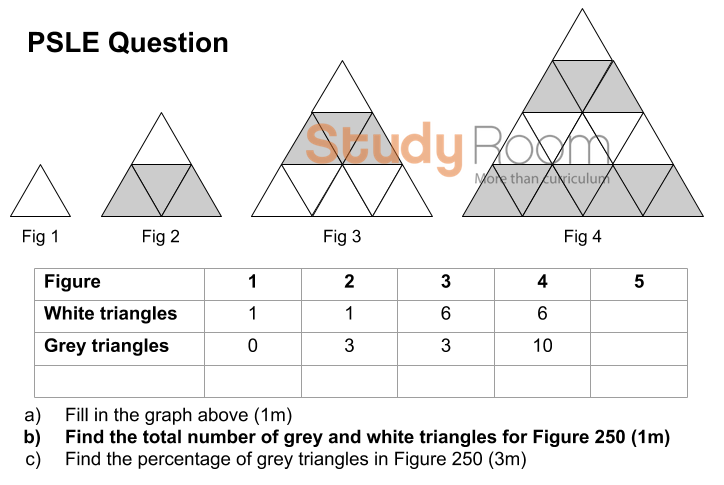# How to solve number pattern problems

In the 2019 PSLE Math exam, a few challenging questions caught the attention of both students and parents.

One number pattern question proved particularly challenging.Solving number patterns step by step:

Question: What is the total number of triangles for Figure 250?

• Drawing more triangles. In this case, it will be impossible to complete – even figure 10 would be an issue, so we need to give up on this brute force method and look for a pattern.
• Instead of drawing, perhaps a table might help. It would be quicker to tabulate than to draw. The table helps with analysis and for us to see a pattern. You may fill in more rows/columns to better help you identify a pattern.
 Figure Total Number of Triangles Figure 1 1 Figure 2 4 Figure 3 9 Figure 4 16 Figure 5 ?

• Finding a pattern. The pattern should be generalised so that we can find the solution for any figure number without knowing the prior figure. For example, one pattern that students may find is: 1+3 = 4, 4+5 = 9, 9+7 = 16. This is a pattern that still depends on the previous figure, so it is not possible to solve for Figure 250 unless you know the number for Figure 249.
• Try and try again. We need to persevere and try various methods! Notice that the triangles get bigger not only in length but also height. This is an important insight – eventually we find the pattern: For Figure 5, the pattern is 5 x 5 = 25 triangles.

And for Figure 250, the pattern is 250 x 250 = 62500 triangles.

Extending the learning

At Study Room, we do not just stop there. To ensure our students understand and internalize the concepts, we extend their learning by tweaking the question. An example could be another question such as:

Can you find the total number of triangles for figure 300?

 Figure Total Number of Triangles Figure 1 9 Figure 2 16 Figure 3 25

The correct answer (91204). I hope you got it right!